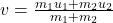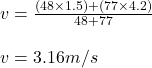## After skiding down a snow-covered hill on an inner tube, Ashley is coasting across a level snowfield at a constant velocity of +1.5 m/s. Mir

Question

After skiding down a snow-covered hill on an inner tube, Ashley is coasting across a level snowfield at a constant velocity of +1.5 m/s. Miranda runs after her at a velocity of +4.2 m/s and hops on the inner tube. How fast do the two of them slide across the snow together on the inner tube? Ashley’s mass is 48 kg, and Miranda’s is 77 kg. Ignore the mass of the inner tube and any friction between the inner tube and the snow.

in progress 0
6 months 2021-08-09T20:05:09+00:00 2 Answers 0 views 0

## Answers ( )

Explanation:

Mass of Ashley = 48 kg

Mass of Miranda = 77 kg

u1 = 1.5 m/s

u2 = 4.2 m/s

m1u1 + m2u2 = (m1 + m2)v

v = m1u1 + m2u2 / m1 + m2

So v = (48×1.5 + 77×4.2) / (48+77)

= 3.1632

= 3.16m/s

= 3.16m/s

Explanation:

Mass of Ashley m₁ = 48kg

Mass of Miranda m₂ = 77kg

Initial velocity of Ashley u₁ = 1.5m/s

Initial velocity of Miranda u₂ = 4.2m/s

Let v be the velocity of the object after collision

m₁u₁ + m₂u₂ = (m₁ + m₂)v

substitute the values

m₁ = 48kg, m₂ = 77kg,  u₁ = 1.5m/s,  u₂ = 4.2m/s